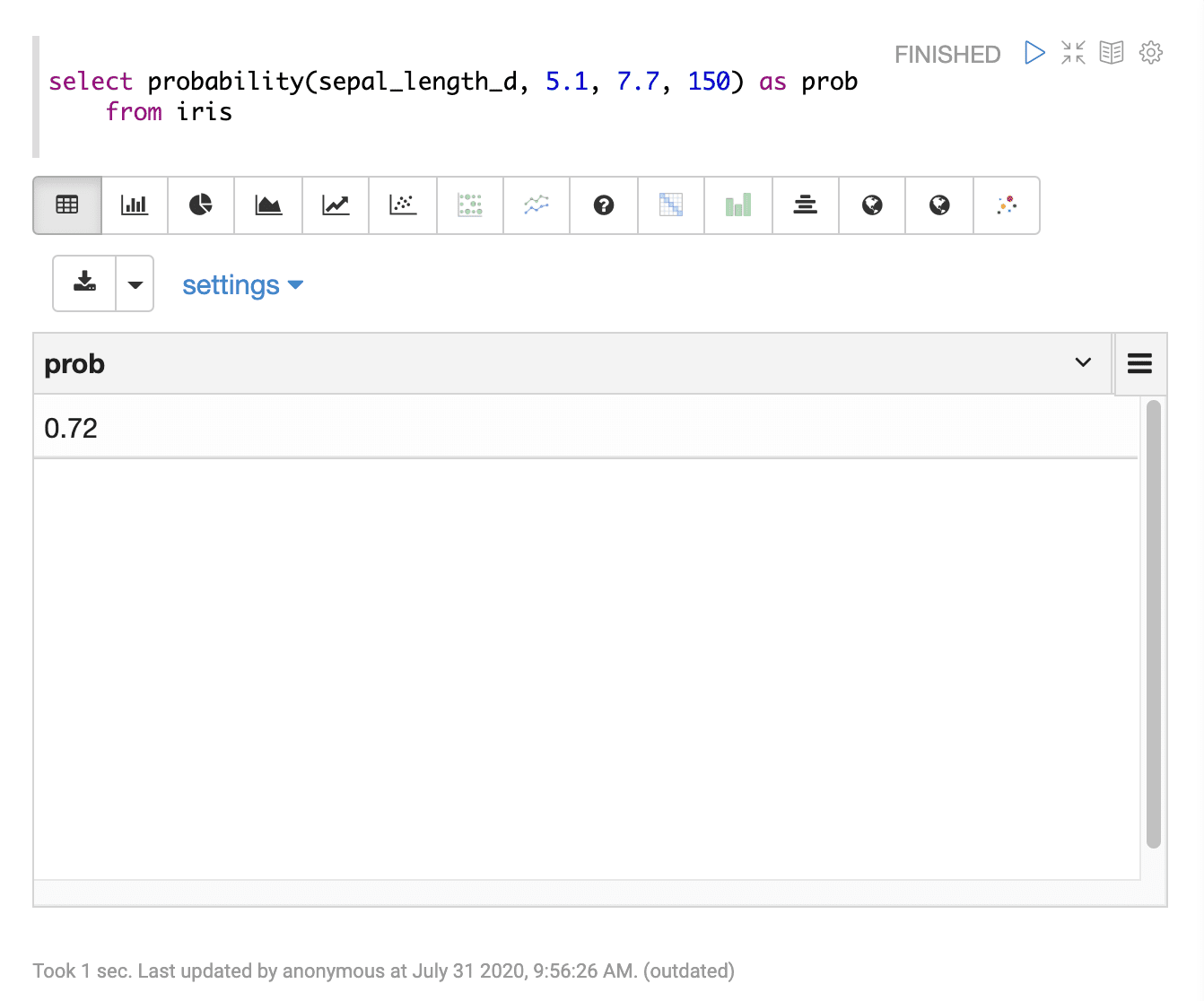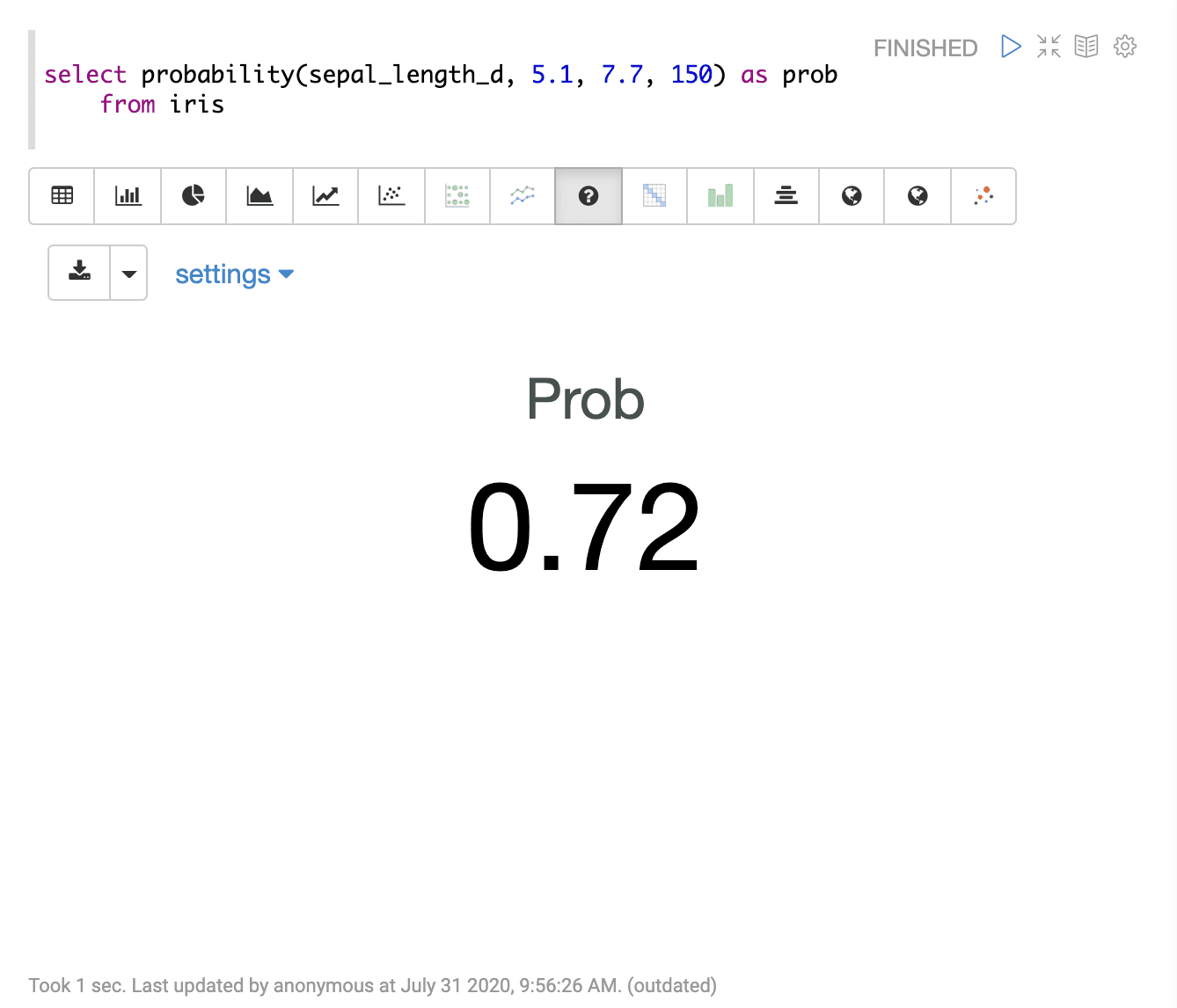# Probability (probability)

The `probability` function calculates the probability that a value drawn from a numeric field will fall within specific range. The `probability` function takes four parameters:

1. the numeric field to compute the probability from

2. the numeric start range

3. the numeric end range

4. the sample size

## Sample syntax

``````select probability(sepal_length_d, 5.1, 7.7, 150) as prob
from iris``````

## Result set

The result set for the `probability` function contains a single record with the calculated probability. The `probability` function returns the probability calculation.

Sample result set in Apache Zeppelin## Visualization

Sample visualization of the probability function using the Apache Zeppelin number visualization: